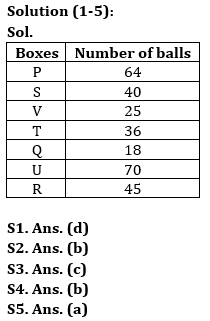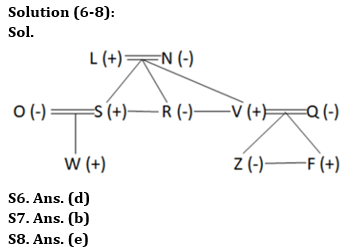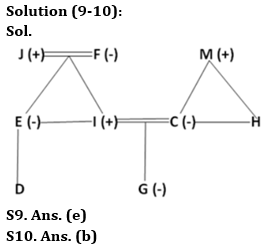Latest Banking jobs   »

# Reasoning Quiz For Bank of Baroda SO 2023 -22nd May

Directions (1-5): Study the following information carefully and answer the questions given below:
Seven boxes are placed one above the other. Each box contains different numbers of balls-18, 25, 36, 40, 45, 64 and 70 (but not necessary in the same order).
Box U is kept below box S. Two boxes are kept between box P and the box containing 36 balls which is kept below Box P. Box T contains double number of balls than box Q. Box R contains 5 balls more than box S and is not kept at the top. The number of balls in box V is a square of a number. Only one box is kept between box containing 36 balls and 40 balls. Five boxes are kept between box containing 64 balls and Box R. Box V is immediately above box T. Three boxes are kept between box S and box U.

Q1. How many boxes are placed above the box which contain 70 balls?
(a) Three
(b) One
(c) Two
(d) More than four
(e) None

Q2. Which of the following box is placed immediate below box Q?
(a) V
(b) The box which contain maximum number of balls
(c) R
(d) The box which contain 36 balls
(e) None of these

Q3. What is the difference between number of balls in box V and box Q?
(a) 20
(b) 15
(c) 7
(d) 10
(e) None of these

Q4. Which of the following is not true about box R?
(a) Box R is placed at bottom
(b) Box T contain 12 balls less than R
(c) Box R contain 45 balls
(d) Only three boxes are placed between R and V
(e) Both (b) and (d)

Q5. Which of the following box contain second lowest number of balls?
(a) V
(b) S
(c) T
(d) Q
(e) None of these

Directions (6-8): Study the information carefully and answer the questions accordingly.
There are ten members in a family of three generations with three married couples. R is the aunt of W and is unmarried. V is married to Q who is the daughter-in-law of L. N is the mother of S who is the brother of R. F is the nephew of S. V is the father of Z who is the granddaughter of L. W is the son of O. N has three children.

Q6. Who is the mother of F?
(a) Wife of L
(b) Wife of S
(c) Sister of S
(d) Q
(e) None of these

Q7. How L is related to S?
(a) Grandfather
(b) Father
(c) Uncle
(d) Nephew
(e) None of these

Q8. Four from the following five are similar in a certain way and forms a group. Find out the one who does not belong to that group.
(a) L
(b) W
(c) F
(d) V
(e) Z

Directions (9-10): Study the following information carefully and answer the questions accordingly.
A family has nine members with two married couples. C is the wife of I. F is the mother of E. M is the grandfather of G. E is the mother of D. G is the daughter of C who is the sister of H. E is the only sister of I. J is the father of E.

Q9. How is D related to J?
(a) Granddaughter
(b) Grandson
(c) Daughter
(d) Son
(e) Can’t be determined

Q10. Who is the father of H?
(a) F
(b) M
(c) J
(d) D
(e) Can’t be determined

Solutions## FAQs

### What is the selection process of Bank of Baroda AO 2023?

The selection process of Bank of Baroda AO consists of Online exam and Interview

#### Congratulations!Union Budget 2023-24: Free PDF